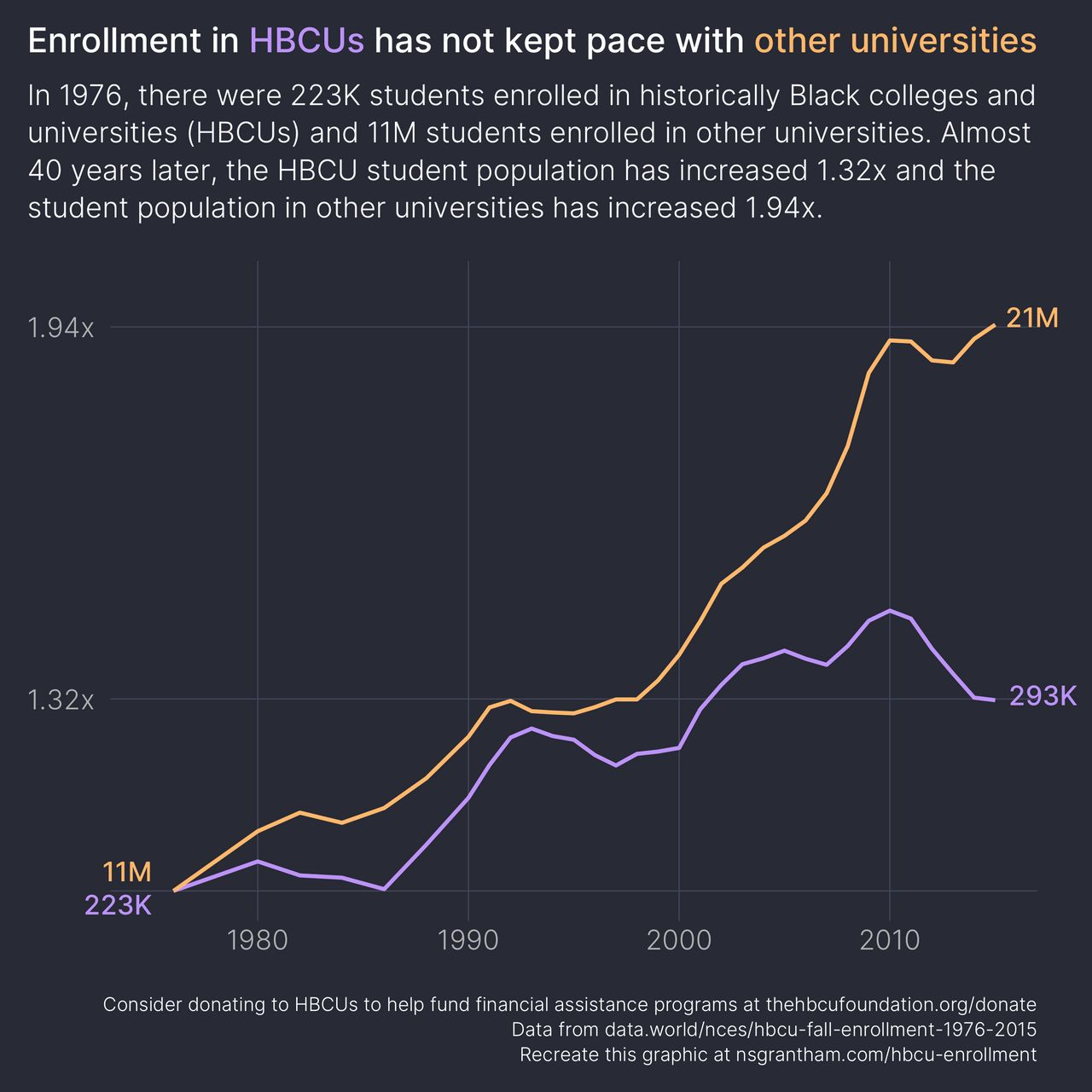# Student Enrollment at HBCUs

February 21, 2021
Medium: R and ggplot2
Large: JPEG
``````library(tidyverse)
library(stringr)
library(ggtext)
library(ggdark)

hbcu <- read_csv("https://raw.githubusercontent.com/rfordatascience/tidytuesday/master/data/2021/2021-02-02/hbcu_all.csv") %>%
select(year = Year, hbcu_enrollment = `Total enrollment`)

# https://nces.ed.gov/programs/digest/d13/tables/dt13_303.10.asp
univ_wide <- read_xls("tabn303.10.xls", sheet = 1, range = "A6:B93", col_names = FALSE) %>%
rename(year = `...1`, total_enrollment = `...2`) %>%
filter(!is.na(year)) %>%
mutate(
year = str_replace_all(year, "\\.", ""),
year = str_replace(year, "\\\\1\\\\", ""),
year = str_replace(year, "\\\\5\\\\", ""),
year = as.integer(str_trim(year)),
total_enrollment = as.integer(total_enrollment)
) %>%
left_join(hbcu, by = "year") %>%
filter(!is.na(hbcu_enrollment)) %>%
mutate(non_hbcu_enrollment = total_enrollment - hbcu_enrollment) %>%
select(-total_enrollment)

univ_wide %>%
filter(year %in% c(1976, 2015))
``````
``````# A tibble: 2 x 3
year hbcu_enrollment non_hbcu_enrollment
<dbl>           <dbl>               <dbl>
1  1976          222613            10789524
2  2015          293388            20972612
``````
``````univ_long <- univ_wide %>%
mutate(
hbcu_multiple = hbcu_enrollment / 222613,
non_hbcu_multiple = non_hbcu_enrollment / 10789524
) %>%
select(year, hbcu_multiple, non_hbcu_multiple) %>%
pivot_longer(
hbcu_multiple:non_hbcu_multiple,
names_to = "type",
values_to = "value"
)

non_hbcu_color <- "#ffb86c"
hbcu_color <- "#bd93f9"

ggplot(univ_long, aes(year, value, group = type, color = type)) +
geom_line(size = 1) +
annotate("text", x = 1975, y = 1.00, hjust =  1.0, vjust = -0.5, label =  "11M", size = 5, family = "Inter-Medium", color = non_hbcu_color) +
annotate("text", x = 1975, y = 1.00, hjust =  1.0, vjust =  1.2, label = "223K", size = 5, family = "Inter-Medium", color = hbcu_color) +
annotate("text", x = 2015, y = 1.94, hjust = -0.2, vjust =  0.0, label =  "21M", size = 5, family = "Inter-Medium", color = non_hbcu_color) +
annotate("text", x = 2015, y = 1.31, hjust = -0.2, vjust =  0.0, label = "293K", size = 5, family = "Inter-Medium", color = hbcu_color) +
coord_cartesian(clip = "off") +
scale_color_manual(values = c(hbcu_color, non_hbcu_color)) +
scale_y_continuous(breaks = c(1, 1.32, 1.94), limits = 1:2, labels = c("", "1.32x", "1.94x")) +
labs(
title = glue::glue("Enrollment in <span style='color:{hbcu_color};font-family:\"Inter-Medium\";'>HBCUs</span> has not kept pace with <span style='color:{non_hbcu_color};font-family:\"Inter-Medium\";'>other universities</span>"),
subtitle = "In 1976, there were 223K students enrolled in historically Black colleges and\nuniversities (HBCUs) and 11M students enrolled in other universities. Almost\n40 years later, the HBCU student population has increased 1.32x and the\nstudent population in other universities has increased 1.94x.",
caption = "Consider donating to HBCUs to help fund financial assistance programs at thehbcufoundation.org/donate\nData from data.world/nces/hbcu-fall-enrollment-1976-2015\nRecreate this graphic at nsgrantham.com/hbcu-enrollment",
x = NULL,
y = NULL
) +
guides(color = FALSE) +
dark_theme_minimal(base_family = "Inter-Light", base_size = 18) +
theme(
plot.title = element_markdown(family = "Inter-Medium", size = 18, margin = margin(0, 0, 0.9, 0, unit = "line")),
plot.title.position = "plot",
plot.subtitle = element_text(size = 15, lineheight = 1.1, margin = margin(0, 0, 1.2, 0, unit = "line")),
plot.caption = element_text(size = 10, lineheight = 1.1),
plot.caption.position = "plot",
plot.margin = margin(1, 2, 1, 1, unit = "line"),
plot.background = element_rect(color = "#282a36", fill = "#282a36"),
panel.grid.major.x = element_line(color = "#44475a", size = 0.3),
panel.grid.major.y = element_line(color = "#44475a", size = 0.3),
panel.grid.minor.x = element_blank(),
panel.grid.minor.y = element_blank(),
axis.text.x = element_text(margin = margin(0, 0, 1, 0, unit = "line"))
)

ggsave("hbcu-enrollment.png", width = 8, height = 8)
``````